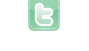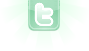## Using a variable which is defined in actor in a world

I have kind of a counter in an actor and when it hits a ceirtain case, the variable which is defiened in the actor should = 1. When the Variable == 0 the world should delete the actor and create a different one. How can I ask in the World if the variable is == 0?
FarbKlexx wrote...
I have kind of a counter in an actor and when it hits a ceirtain case, the variable which is defiened in the actor should = 1. When the Variable == 0 the world should delete the actor and create a different one. How can I ask in the World if the variable is == 0?
Instead of asking, just tell the world when it hits zero. In actor:
`if (counter > 0 && --counter == 0) ((MyWorld)getWorld()).doSomething();`
```public void doSomething()